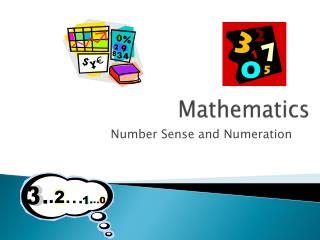DownloadDownload PresentationMathematics

# Mathematics

Download Presentation## Mathematics

- - - - - - - - - - - - - - - - - - - - - - - - - - - E N D - - - - - - - - - - - - - - - - - - - - - - - - - - -
##### Presentation Transcript

1. Mathematics Number Sense and Numeration

2. Grade 8: • represent, compare, and order equivalent representations of numbers, including those involving positive exponents; • solve problems involving whole numbers, decimal numbers, fractions, and integers, using a variety of computational strategies; • solve problems by using proportional reasoning in a variety of meaningful contexts.

3. BIG Ideas: • There are many ways to represent numbers • Numbers tell how much or how many • Number benchmarks are useful ( 10,25,100,1000) • We can draw conclusions about numbers by classifying them – how many digits, odd/even etc. • Patterns in place values make it easier to operate and work with numbers • It is important to know when to use each operation ( addition, subtraction, multiplication or division) • There are many ways to add, subtract, multiply or divide numbers • It is important to take advantage of and use the relationships between numbers.

4. Quantity Relationships

5. Exponential Notation • express repeated multiplication using exponential notation e.g., 2 x 2 x 2 x 2 = 24

6. Powers of Ten • represent whole numbers in expanded form using powers of ten (e.g., 347 = 3 x 102 + 4 x 101 + 7)

7. Rational Numbers • represent, compare, and order rational numbers (i.e., positive and negative fractions and decimals to thousandths

8. Equivalent Forms • translate between equivalent forms of a number (i.e., decimals, fractions, percents)

9. Factors and Common Multiples • determine common factors and common multiples using the prime factorization of numbers (e.g., the prime factorization of 12 is 2 x 2 x 3; the prime factorization of 18 is 2 x 3 x 3; the greatest common factor of 12 and 18 is 2 x 3 or 6; the least common multiple of 12 and 18 is 2 x 2 x 3 x 3 or 36).

10. Operational Sense

11. Multi-Step Problems • solve multi-step problems arising from real-life contexts and involving whole numbers and decimals, using a variety of tools (e.g., graphs, calculators) and strategies (e.g., estimation, algorithms)

12. Order of Operations -BEDMAS • evaluate expressions that involve integers, including expressions that contain brackets and exponents, using order of operations B- brackets E -exponents D- division M-multiplication A -addition S - subtraction

13. Decimal Numbers • multiply and divide decimal numbers by various powers of ten (e.g., "To convert 230 000 cm3 to cubic metres, I calculated in my head 230 000 ÷ 106 to get 0.23 m3.”) Sample problem: Use a calculator to help you generalize a rule for dividing numbers by 1 000 000.)

14. Square Roots Estimate, and verify using a calculator, the positive square roots of whole numbers, and distinguish between whole numbers that have whole-number square roots (i.e., perfect square numbers) and those that do not Sample problem: Explain why a square with an area of 20 cm2 does not have a whole-number side length.).

15. Percents Solve problems involving percents expressed to one decimal place (e.g.,12.5%) and whole-number percents greater than 100 (e.g., 115%) Sample problem: The total cost of an item with tax included [115%] is \$23.00. Use base ten materials to determine the price before tax.

16. Estimation • use estimation when solving problems involving operations with whole numbers, decimals, percents, integers, and fractions, to help judge the reasonableness of a solution;

17. Fractions • represent the multiplication and division of fractions, using a variety of tools and strategies (e.g., use an area model to solve problems involving addition, subtraction, multiplication, and division with simple fractions;

18. Integers • represent the multiplication and division of integers, using a variety of tools [e.g., if black counters represent positive amounts and red counters represent negative amounts, you can model 3 x (–2) as three groups of two red counters]; • solve problems involving operations with integers, using a variety of tools (e.g., two colour counters, virtual manipulatives, number lines)

19. Proportional Relationships

20. Real-life SituationsInvolving Two Quantities • identify and describe real-life situations involving two quantities that are directly proportional (e.g., the number of servings and the quantities in a recipe, mass and volume of a substance, circumference and diameter of a circle.

21. problems involving proportions solve problems involving proportions,using concrete materials, drawings, and variables Sample problem: The ratio of stone to sand in HardFast Concrete is 2 to 3. How much stone is needed if 15 bags of sand are used?

22. Percent • solve problems involving percent that arise from real-life contexts (e.g., discount, sales tax, simple interest) Sample problem: In Ontario, people often pay a provincial sales tax [PST] of 8% and a federal sales tax [GST] of 7% when they make a purchase. Does it matter which tax is calculated first? Explain your reasoning.

23. Rates • solve problems involving rates Sample problem: A pack of 24 CDs costs \$7.99. A pack of 50 CDs costs \$10.45. What is the most economical way to purchase 130 CDs?